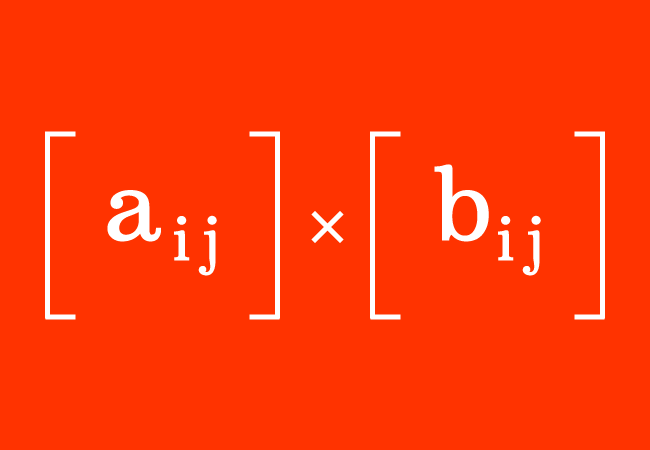# Matrix multiplication

A mathematical operation of multiplying a matrix by another matrix is called the multiplication of the matrices.

## Introduction

In matrices, the entries (or elements) are arranged in rows and columns. It confuses us how to multiply two or more matrices mathematically when the matrices participate in multiplication. However, there is a systematic procedure for multiplying the matrices. So, let’s learn how to multiply the matrices in mathematics.

### ConditionThe multiplication of the matrices is possible if the total number of elements in a row of the first matrix is equal to the total number of entries in a column of the second matrix.

$A \,=\, \big[a_{ij}\big]_{m \times r}$ and $B \,=\, \big[b_{ij}\big]_{r \times n}$

$A$ and $B$ are two matrices. The matrix $A$ is a matrix of the order $m \times r$ and the matrix $B$ is a matrix of the order $r \times n$. The $a_{ij}$ and $b_{ij}$ represent each entry in the matrices $A$ and $B$ respectively. Here, $i$ and $j$ represent “the number of the row” and “the number of the column” of the element.

The multiplication of the matrices is written in mathematics as follows.

$\implies$ $A \times B$ $\,=\,$ $\big[a_{ij}\big]_{m \times r}$ $\times$ $\big[b_{ij}\big]_{r \times n}$

Let’s assume that C is a matrix, which represents the product of the matrices $A$ and $B$.

$\,\,\,\therefore\,\,\,\,\,\,$ $C$ $\,=\,$ $\big[c_{ij}\big]_{m \times n}$

The number of rows in the matrix $C$ is equal to the number of the rows of the matrix $A$. Similarly, the number of columns in the matrix $C$ is equal to the number of the columns of the matrix $B$. Hence, the product of the matrices $A$ and $B$ is the matrix $C$ of the order $m \times n$.

This algebraic analysis can be understood much clear in the below examples section.

### Procedure

There are two simple steps for multiplying the matrices in mathematics.

1. Multiply the entries in each column of the second matrix by the elements in every row of the first matrix respectively.
2. Add the products of the entries to evaluate the elements for the matrix that represents the multiplication of the matrices.

### Examples

Let’s learn how to multiply the matrices from the following two examples.

$(1).\,\,\,$ ${\begin{bmatrix} 1 & 2 \\ 3 & 4 \\ \end{bmatrix}}$ $\times$ ${\begin{bmatrix} 5 & 6 \\ 7 & 8 \\ \end{bmatrix}}$

$\implies$ $\begin{bmatrix} 1 & 2 \\ 3 & 4 \\ \end{bmatrix}$ $\times$ $\begin{bmatrix} 5 & 6 \\ 7 & 8 \\ \end{bmatrix}$ $\,=\,$ $\begin{bmatrix} 1 \times 5+2 \times 7 & 1 \times 6+2 \times 8 \\ 3 \times 5+4 \times 7 & 3 \times 6+4 \times 8 \\ \end{bmatrix}$ $\,=\,$ $\begin{bmatrix} 5+14 & 6+16 \\ 15+28 & 18+32 \\ \end{bmatrix}$

$\,\,\,\therefore\,\,\,\,\,\,$ $\begin{bmatrix} 1 & 2 \\ 3 & 4 \\ \end{bmatrix}$ $\times$ $\begin{bmatrix} 5 & 6 \\ 7 & 8 \\ \end{bmatrix}$ $\,=\,$ $\begin{bmatrix} 19 & 22 \\ 43 & 50 \\ \end{bmatrix}$

The order of each multiplying matrix is $2$ in this example. Hence, the order of their product matrix is also $2 \times 2$.

$(2).\,\,\,$ ${\begin{bmatrix} 1 & 2 & 3 \\ \end{bmatrix}}$ $\times$ ${\begin{bmatrix} 4 & 5 \\ 6 & 7 \\ 8 & 9 \\ \end{bmatrix}}$

$\implies$ ${\begin{bmatrix} 1 & 2 & 3 \\ \end{bmatrix}}$ $\times$ ${\begin{bmatrix} 4 & 5 \\ 6 & 7 \\ 8 & 9 \\ \end{bmatrix}}$ $\,=\,$ ${\begin{bmatrix} 1 \times 4+2 \times 6+3 \times 8 & 1 \times 5+2 \times 7+3 \times 9\\ \end{bmatrix}}$

$\implies$ ${\begin{bmatrix} 1 & 2 & 3 \\ \end{bmatrix}}$ $\times$ ${\begin{bmatrix} 4 & 5 \\ 6 & 7 \\ 8 & 9 \\ \end{bmatrix}}$ $\,=\,$ ${\begin{bmatrix} 4+12+24 & 5+14+27\\ \end{bmatrix}}$

$\,\,\,\therefore\,\,\,\,\,\,$ ${\begin{bmatrix} 1 & 2 & 3 \\ \end{bmatrix}}$ $\times$ ${\begin{bmatrix} 4 & 5 \\ 6 & 7 \\ 8 & 9 \\ \end{bmatrix}}$ $\,=\,$ ${\begin{bmatrix} 40 & 46\\ \end{bmatrix}}$

The order of the first matrix is $1 \times 3$ and the order of the second matrix is $3 \times 2$. Hence, the order of their product matrix is $1 \times 2$.

#### Problems

List of the practice questions on matrix multiplication with solutions to learn how to multiply the matrices of the same order or different order in mathematics.

Latest Math Topics
Jun 26, 2023
Jun 23, 2023

Latest Math Problems
Jul 01, 2023
Jun 25, 2023
###### Math Questions

The math problems with solutions to learn how to solve a problem.

Learn solutions

Practice now

###### Math Videos

The math videos tutorials with visual graphics to learn every concept.

Watch now

###### Subscribe us

Get the latest math updates from the Math Doubts by subscribing us.